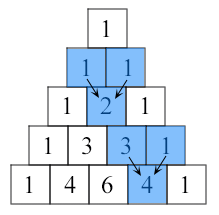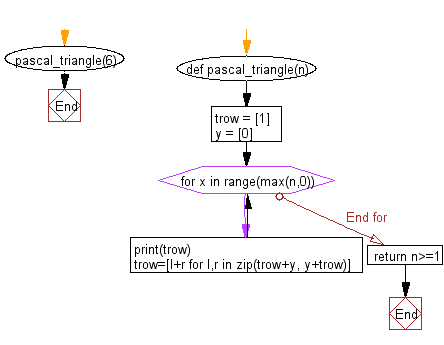﻿ Python Exercise: Print out the first n rows of Pascal's triangle - w3resource# Python Exercise: Print out the first n rows of Pascal's triangle

## Python Functions: Exercise-13 with Solution

Write a Python function that prints out the first n rows of Pascal's triangle.

Note : Pascal's triangle is an arithmetic and geometric figure first imagined by Blaise Pascal.

Sample Pascal's triangle :Each number is the two numbers above it added together

Sample Solution :-

Python Code :

``````def pascal_triangle(n):
trow = 
y = 
for x in range(max(n,0)):
print(trow)
trow=[l+r for l,r in zip(trow+y, y+trow)]
return n>=1
pascal_triangle(6)
```
```

Sample Output:

```
[1, 1]
[1, 2, 1]
[1, 3, 3, 1]
[1, 4, 6, 4, 1]
[1, 5, 10, 10, 5, 1]
```

Flowchart:## Visualize Python code execution:

The following tool visualize what the computer is doing step-by-step as it executes the said program:

Python Code Editor:

Have another way to solve this solution? Contribute your code (and comments) through Disqus.

What is the difficulty level of this exercise?

Test your Programming skills with w3resource's quiz.

﻿

## Python: Tips of the Day

Decapitalizes the first letter of a string:

Example:

```def tips_decapitalize(s, upper_rest=False):
return s[:1].lower() + (s[1:].upper() if upper_rest else s[1:])
print(tips_decapitalize('PythonTips'))
print(tips_decapitalize('PythonTips', True))
```

Output:

```pythonTips
pYTHONTIPS
```